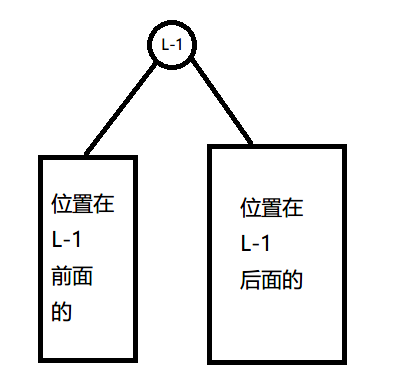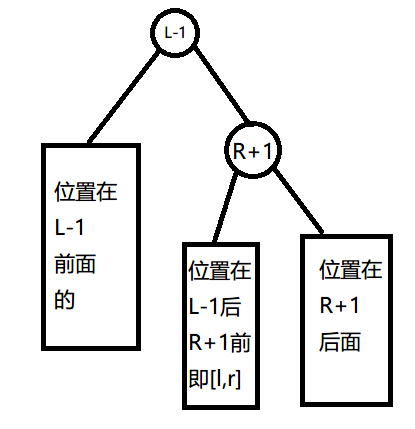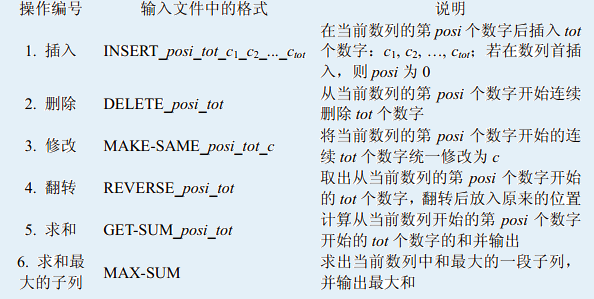# 如何维护区间<h1>「BZOJ1500」「NOI2005」维修数列</h1><h2>Insert 操作</h2>

<h2>Delete 操作</h2>

<h2>MAKE-SAME 操作</h2>

<h2>翻转</h2>

<h2>求和</h2>

<h2>求最大子列</h2>

pushup 的时候分别维护即可，可以 $O(1)$ 维护。

#include <algorithm>
#include <iostream>
#include <cstring>
#include <climits>
#include <cstdio>
#include <vector>
#include <cstdlib>
#include <ctime>
#include <cmath>
#include <queue>
#include <stack>
#include <map>
#include <set>

#define fi first
#define lc (ch[x])
#define se second
#define U unsigned
#define rc (ch[x])
#define Re register
#define LL long long
#define MP std::make_pair
#define CLR(i,a) memset(i,a,sizeof(i))
#define FOR(i,a,b) for(Re int i = a;i <= b;++i)
#define ROF(i,a,b) for(Re int i = a;i >= b;--i)
#define SFOR(i,a,b,c) for(Re int i = a;i <= b;i+=c)
#define SROF(i,a,b,c) for(Re int i = a;i >= b;i-=c)
#define DEBUG(x) std::cerr << #x << '=' << x << std::endl

const int MAXN = 500000+5;

int f[MAXN],ch[MAXN],size[MAXN],val[MAXN],sum[MAXN],ls[MAXN],rs[MAXN],max[MAXN];
int rev[MAXN],tag[MAXN];

int a[MAXN],bin[MAXN],cnt,top,N,M;

inline void pushup(int x){
size[x] = size[lc] + size[rc] + 1;
sum[x] = sum[lc] + sum[rc] + val[x];
max[x] = std::max(std::max(max[lc],max[rc]),rs[lc]+ls[rc]+val[x]);
ls[x] = std::max(ls[lc],sum[lc]+val[x]+ls[rc]);
rs[x] = std::max(rs[rc],sum[rc]+val[x]+rs[lc]);
}

inline void pushdown(int x){
if(tag[x]){
tag[x] = rev[x] = false;
if(lc){
tag[lc] = true;val[lc] = val[x];sum[lc] = val[x]*size[lc];
}
if(rc){
tag[rc] = true;val[rc] = val[x];sum[rc] = val[x]*size[rc];
}
if(val[x] >= 0){
if(lc) ls[lc] = rs[lc] = max[lc] = sum[lc];
if(rc) ls[rc] = rs[rc] = max[rc] = sum[rc];
}
else{
if(lc) ls[lc] = rs[lc] = 0,max[lc] = val[x];
if(rc) ls[rc] = rs[rc] = 0,max[rc] = val[x];
}
}
if(rev[x]){
rev[x] = 0;rev[lc] ^= 1;rev[rc] ^= 1;
std::swap(ls[lc],rs[lc]);std::swap(ls[rc],rs[rc]);
std::swap(ch[lc],ch[lc]);std::swap(ch[rc],ch[rc]);
}
}

inline void rotate(int x){
int y = f[x],z = f[y],k = ch[y] == x,w = ch[x][!k];
ch[z][ch[z] == y] = x;f[x] = z;
ch[x][!k] = y;f[y] = x;
ch[y][k] = w;f[w] = y;
pushup(y);pushup(x);
}
int root;
inline void splay(int x,int v){
int y,z;
while((y = f[x]) != v){
if((z = f[y]) != v) rotate((ch[z] == y)^(ch[y] == x) ? x : y);
rotate(x);
}
if(!v) root = x;
}

int getkth(int rk){
int x = root;
while(233){
pushdown(x);
if(rk <= size[lc]) x = lc;
else if(rk == size[lc]+1) return x;
else rk -= size[lc]+1,x = rc;
}
}

inline int build(int l,int r,int fa){
if(l > r) return 0;
int mid = (l + r) >> 1,x;
x = top ? bin[top--] : ++cnt;
f[x] = fa;val[x] = a[mid];
rev[x] = tag[x] = 0;
lc = build(l,mid-1,x);rc = build(mid+1,r,x);
pushup(x);return x;
}

inline void insert(int l,int tot){
int r = l+1;
l = getkth(r);r = getkth(r+1);
splay(l,0);splay(r,l);
FOR(i,1,tot) scanf("%d",a+i);
ch[r] = build(1,tot,r);
N += tot;pushup(r);pushup(l);
}

inline void erase(int x){
if(!x) return;
bin[++top] = x;
erase(lc);erase(rc);
}

inline void del(int l,int r){
N -= r-l+1;
l = getkth(l);r = getkth(r+2);
splay(l,0);splay(r,l);
erase(ch[r]);ch[r] = 0;
pushup(r);pushup(l);
}

inline void modify(int l,int r,int v){
l = getkth(l);r = getkth(r+2);
splay(l,0);splay(r,l);
int x = ch[r];
val[x] = v;sum[x] = v*size[x];
if(v <= 0) ls[x] = rs[x] = 0,max[x] = v;
else ls[x] = rs[x] = max[x] = sum[x];
tag[x] = 1;
pushup(r);pushup(l);
}

inline void reverse(int l,int r){
l = getkth(l);r = getkth(r+2);
splay(l,0);splay(r,l);
int x = ch[r];
if(!tag[x]){
rev[x] ^= 1;std::swap(lc,rc);std::swap(ls[x],rs[x]);
pushup(r);pushup(l);
}
}

inline int querysum(int l,int r){
l = getkth(l);r = getkth(r+2);
splay(l,0);splay(r,l);
return sum[ch[r]];
}

inline int calc(){
int l = getkth(1),r = getkth(N+2);
splay(l,0);splay(r,l);
return max[ch[r]];
}

int main(){
scanf("%d%d",&N,&M);
max = a = a[N+2] = INT_MIN;
FOR(i,1,N) scanf("%d",a+i+1);
root = build(1,N+2,0);
while(M--){
char opt;
int x,y,z;
scanf("%s",opt);
if(opt == 'I'){
scanf("%d%d",&x,&y);
insert(x,y);
}
if(opt == 'D'){
scanf("%d%d",&x,&y);
del(x,x+y-1);
}
if(opt == 'M' && opt == 'K'){
scanf("%d%d%d",&x,&y,&z);
modify(x,x+y-1,z);
}
if(opt == 'R'){
scanf("%d%d",&x,&y);
reverse(x,x+y-1);
}
if(opt == 'G'){
scanf("%d%d",&x,&y);
printf("%d\n",querysum(x,x+y-1));
}
if(opt == 'M' && opt == 'X'){
printf("%d\n",calc());
}
}
return 0;
}
Last modification：April 7th, 2020 at 11:42 pm